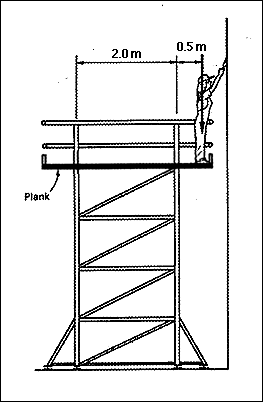## Lecture 14Example Problem

FBD, Components of Reactions
Given in the illustration below of a painter (with a mass of 70 kg) standing on the edge of an elevated plaform. Determine the plank support reactions if the plank she is standing on weighs 8kg.First.... convert to forces!
Wt x 9.81 = newtons

HOW BIG IS one NEWTON?
about the force exerted on your head when one apple falls on it. An item which exerts a force of about 0.5 N is the maximum that a "normal" person can lift. This is approximatly equal to a bag of cement. A small car is about 10 N worth of force.

create FBD
sum moments to determine raction at roller
sum vertical forces to determine second reaction
check